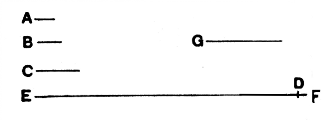#Elements: Book IX, Proposition 20

Math Lair Home > Source Material > Elements > Book IX, Proposition 20

The following is as given in Sir Thomas L. Heath's translation, which can be found in the book The Thirteen Books of The Elements, Vol. 2.

Elements on the Math Lair
Book IBook IIBook IX
Definitions, Postulates, and Common NotionsDefinitionsProposition 20
Proposition 1, Proposition 3, Proposition 14Proposition 36
Proposition 5, Proposition 6,
Proposition 29, Proposition 47

## Proposition 20.

Prime numbers are more than any assigned multitude of prime numbers.

Let A, B, C be the assigned prime numbers;

I say that there are more prime numbers than A, B, C.For let the least number measured by A, B, C be taken,

and let it be DE;
let the unit DF be added to DE.

Then, EF is either prime or not.

First, let it be prime;

then the prime numbers A, B, C, EF have been found which are more than A, B, C.

Next, let EF not be prime;

therefore it is measured by some prime number. [VII. 31]

Let it be measured by the prime number G.

I say that G is not the same with any of the numbers A, B, C.

For, if possible, let it be so.

Now A, B, C measure DE;

therefore G also will measure DE.

But it also measures EF.

Therefore G, being a number, will measure the remainder, the unit DF;

which is absurd.

Therefore G is not the same with an y one of the numbers A, B, C.

And by hypothesis it is prime.

Therefore the prime numbers A, B, C, G have been found which are more than the assigned multitude of A, B, C.

Q.E.D.